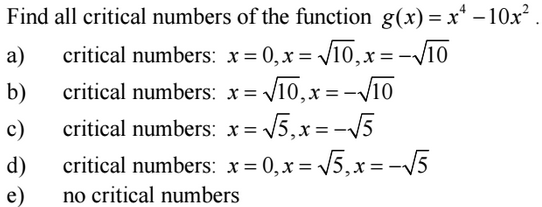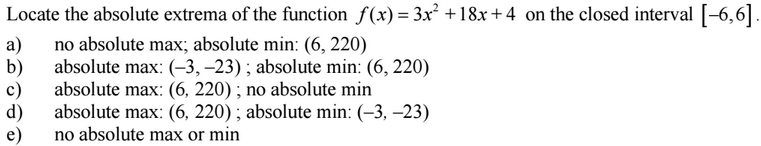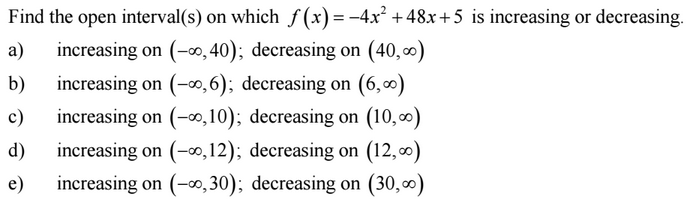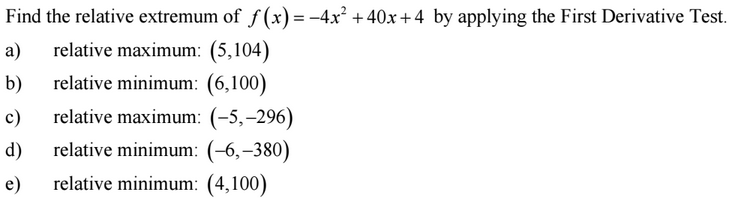### Day 36 - Rolle's Theorem and Mean Value Theorem - 02.24.16

 Update Calculus Equations/Theorems Critical NumbersExtremaIncreasing/Decreasing FunctionsRelative ExtremaReviewPre-calculusExtremaMinimaMaximaAbsolute and Relative Extrema (video)Interval NotationExtrema (video)/Critical Numbers ((checkpoints)How can extrema be defined for a function?How can critical numbers be calculated using derivatives?How are critical numbers related to extrema?How does Extreme Value Theorem work?Increasing/Decreasing Functions (video) (checkpoints)How can derivatives be used to find intervals of increasing and decreasing?How can derivatives be used to find relative extrema?LessonChallenge 3.3Rolle's TheoremSketch a graph of a function on a closed interval [a, b] that:has endpoints with equal y-values.Sketch a graph of a function on a closed interval [a, b] that:has endpoints with equal y-valueshas no point that has a slope of zeroSketch a graph of a function on a closed interval [a, b] that:has endpoints with equal y-valueshas no point that has a slope of zerois continuousSketch a graph of a function on a closed interval [a, b] that:has endpoints with equal y-valueshas no point that has a slope of zerois continuous from [a, b]is differentiable on the interval (a, b)Mean Value TheoremSketch a graph of a function on a closed interval [a, b] that:has endpoints with unequal y-values.Sketch a graph of a function on a closed interval [a, b] that:has endpoints with unequal y-valueshas no points with equal to the slope of points a and bSketch a graph of a function on a closed interval [a, b] that:has endpoints with unequal y-valueshas no points with equal to the slope of points a and bis continuousSketch a graph of a function on a closed interval [a, b] that:has endpoints with unequal y-valueshas no points with equal to the slope of points a and bis continuous from [a, b]is differentiable on the interval (a, b)VideosPracticeCheckpoints Exit Ticket Posted on the board at the end of the block.HomeworkStudy! Help Request List Standard(s) APC.7Analyze the derivative of a function as a function in itself.Includes:comparing corresponding characteristics of the graphs of f, f', and f''​defining the relationship between the increasing and decreasing behavior of f and the sign of f'​translating verbal descriptions into equations involving derivatives and vice versaanalyzing the geometric consequences of the Mean Value Theorem;defining the relationship between the concavity of f and the sign of f"; and ​identifying points of inflection as places where concavity changes and finding points of inflection.APC.8Apply the derivative to solve problems.Includes:​analysis of curves and the ideas of concavity and monotonicityoptimization involving global and local extrema;modeling of rates of change and related rates;use of implicit differentiation to find the derivative of an inverse function;interpretation of the derivative as a rate of change in applied contexts, including velocity, speed, and acceleration; anddifferentiation of nonlogarithmic functions, using the technique of logarithmic differentiation.**AP Calculus BC will also apply the derivative to solve problems.Includes:​analysis of planar curves given in parametric form, polar form, and vector form, including velocity and acceleration vectors;​numerical solution of differential equations, using Euler’s method;​l’Hopital’s Rule to test the convergence of improper integrals and series; and​geometric interpretation of differential equations via slope fields and the relationship between slope fields and the solution curves for the differential equations.